Click to Chat

1800-1023-196

+91-120-4616500

CART 0

• 0

MY CART (5)

Use Coupon: CART20 and get 20% off on all online Study Material

ITEM
DETAILS
MRP
DISCOUNT
FINAL PRICE
Total Price: Rs.

There are no items in this cart.
Continue Shopping```The Modulus and the Conjugate of a Complex Number

Table of Content

What is meant by the modulus of a Complex number?

In Cartesian form

In Polar or trigonometric form

In Exponential form

Explain the properties of Modulus

What does Arg mean in Mathematics?

How do you find the general argument, amplitude and least positive argument?

What are the properties of argument?

How do you find the conjugate of a complex number?

In Cartesian form

In Polar or trigonometric form

In Exponential form

What are the Properties of Conjugate?

What is meant by the Modulus of a Complex Number?

Modulus represents the distance of the point on the argand plane from the origin. For any Complex Number z, its modulus is represented by |z|.

Modulus of any complex number is always positive, i.e. |z| > 0. Also, all the complex numbers having the same modulus lies on a circle.

In Cartesian form

z1 = x + iy complex number in Cartesian form, then its modulus can be found by |z| =Example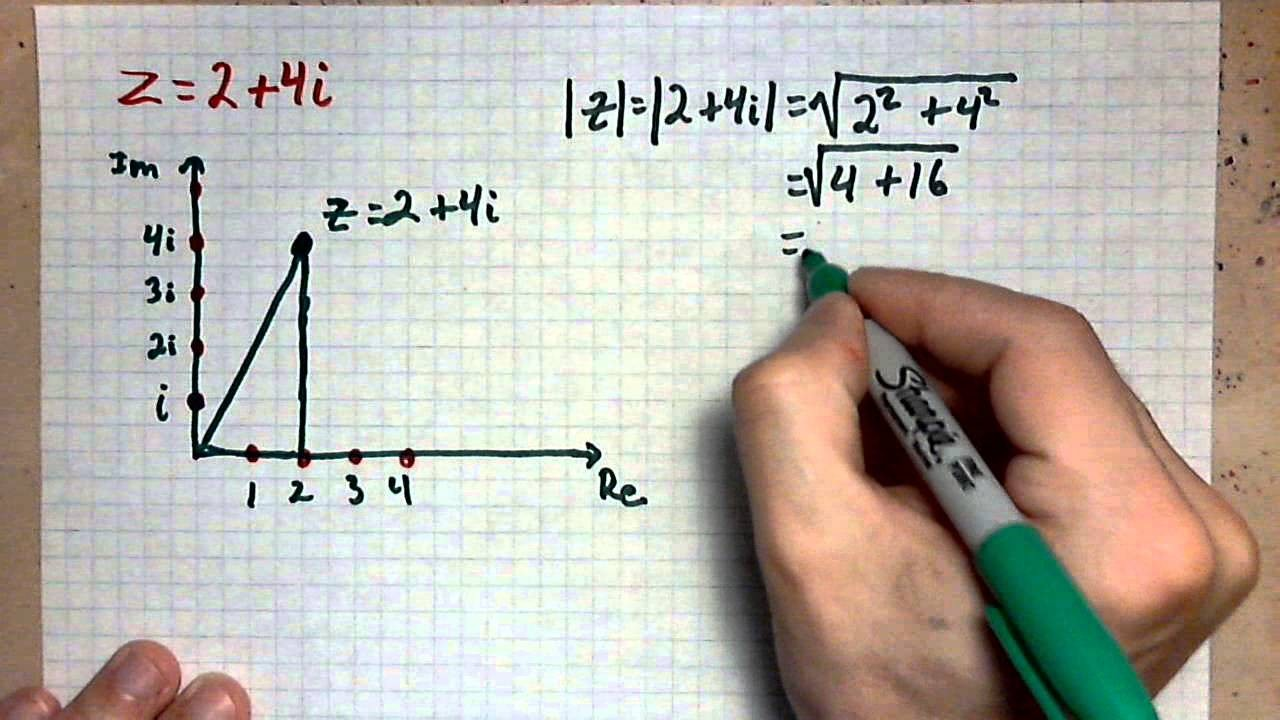In Polar or Trigonometric formAny complex number in polar form is represented by z = r(cos∅ + isin∅) or z = r cis ∅ or z = r∠∅, where r represents the modulus or the distance of the point z from the origin. And ∅ is the angle subtended by z from the positive x-axis.

Here,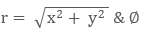represent its argument,

Which we shall discuss in next section.

In Exponential form

Complex number z in exponential form can be expressed asrepresents the modulus and ∅ is its argument.

Explain the Properties of Modulus

All the properties of modulus are listed here below:(such types of Complex Numbers are also called as Unimodular)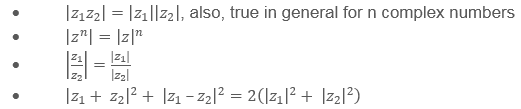This property indicates the sum of squares of diagonals of a parallelogram is equal to sum of squares of its all four sides.

Let A(z1 )=x1+iy1  and B(z2 )=x2+ iy2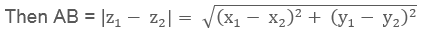|z| = r Represents the locus of circle having centre as origin (0,0) and radius = r.

Similarly, |z – z0|, represents the locus of circle having centre as zo and same radius as r.

If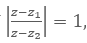where z1 and z2 are two fixed complex numbers, then locus of z is the perpendicular bisectors of line joining z1 and z2.
Triangle Inequalities: | |z1 | - |z2 | | ≤ |z1 ± z2 | ≤ |z1| + |z2|

In the above inequality, first two inequality shows than the absolute value of difference of two sides is always greater than the third side. While the last two inequalities show that the sum of two sides is always greater than third side.

Sign of equality hold if z1, z2  and origin are collinear.

What does Arg mean in Mathematics?

Arg or Argument in complex number represents the angle subtended by any complex point on the argand plane from the positive x –axis. For any complex number z, its argument is represented by arg(z). It gives us the measurement of angle between the positive x-axis and the line joining origin and the point.

We have three ways to express the argument for any complex number.

They are as follows: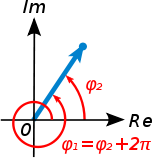General Argument (GA)

For any complex number z = x + iy or z = r(cos⁡∅ + i sin⁡∅),

Re (z) = r cos ⁡∅ and Im(z) = r sin⁡∅

This shows real and imaginary parts of the complex numbers are the function of cosine and sin, and thus they are periodic with the period 2π. That means there exists infinite complex numbers having same angle or argument and same is the case for modulus too.

So, assume any complex number in first quadrant z = x + iy (x, y > 0)

Its General argument will be given bywhere n ∈I.

Here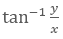is called as the principle value of argument and thus its value will depend upon the quadrant in which the point lies.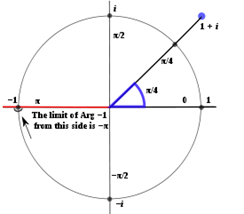Amplitude (amp) or Principle value of Argument (PA)

Because of general argument, a well-defined complex number can’t be expressed. So for the cases where a well-defined complex function is required, we use amplitude or principle value of argument instead of general argument.

In Principle argument, the value of ∅ is restricted to be in the interval  (-π < ∅ ≤ π) or (-π, π]. This range represent the half-circled range from the positive x – axis in the either direction.

Refer the below table to understand the calculation of amplitude of a complex number (z = x + iy) on the basis of different quadrants** General Argument = 2nπ + Principal argument

Least positive argument (LPA)

This is another type of argument, where the range of angle ∅ is kept to be (0 < ∅ ≤ 2π) or (0, 2π]. In this type of argument, only anticlockwise rotation is considered. So for the complex number to be in any quadrant, the angle is calculated from the positive x – axis in the anticlockwise or counter clockwise direction only.

See the below table for the general examples:How do you find the general argument, amplitude and least positive argument?

This can be best understood by taking few examples from different quadrants:** Again here we can note that, General argument = 2nπ + Amplitude

Important Note:

If z is purely Real and positive complex number, then amp(z) = 0

If z is purely Imaginary and positive complex number, then amp(z) = π/2

If z is purely Imaginary and negative complex number, then amp(z) = - π/2

If z is purely Real and negative complex number, then amp(z) = π

What are the properties of argument?

Properties of arguments are as follows:

amp(z1 z2) = amp(z1) + amp(z2)+ 2kπ, k∈I

amp(z1/z2 ) = amp(z1) - amp(z2) + 2kπ, k∈I

amp(zn) = n amp (z) + 2kπ

Above 2kπ, k∈ I is added in RHS and k is choosed such that the value of exponent in RHS belongs to (-π, π]

If amp (z-z0) = ∝ then the locus of z is a ray from zo and making angle of ∝ from positive real axis** Modulus and Argument completely defines a complex number

For zero complex number, that is. z = 0 + i0, Argument is not defined and this is the only complex number which is completely defined only by its modulus that is. |z| = 0.

How do you find the conjugate of a complex number?

Asterisk (symbolically *) in complex number means the complex conjugate of any complex number.

We can also define the complex conjugate of any complex number as the complex number with same real part and same magnitude of imaginary part but with opposite sign as of given complex number.

In Cartesian form

Let z1 = x + iy is any complex number, then its complex conjugate is represented by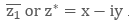Refer the below table to understand it more clearly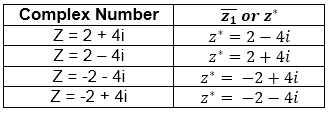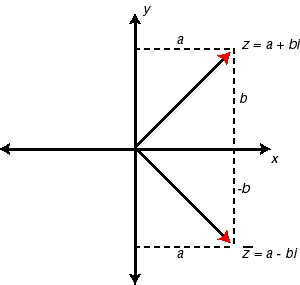In Polar or trigonometric form

Let the complex number in polar form is represented by z = r(cos∅ + isin∅)  or  z = r cis ∅ or z = r ∠∅, then its complex conjugate will be represented by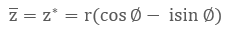In Exponential form

Let z = rei∅ be any complex number written in exponential form, then its complex conjugate can be calculated asWhat are the Properties of Conjugate?

Here are few important properties of conjugate:The Modulus and the Conjugate of a Complex Number
```### Course Features

• 731 Video Lectures
• Revision Notes
• Previous Year Papers
• Mind Map
• Study Planner
• NCERT Solutions
• Discussion Forum
• Test paper with Video Solution